Other Mathematics Commons™

1,158 Full-Text Articles 1,224 Authors 211,409 Downloads97 Institutions

All Articles in Other Mathematics

1,158 full-text articles. Page 8 of 46.

2017Jiangxi Normal University

One Dimensional Complex Ornstein-Uhlenbeck Operator, Yong Chen

Communications on Stochastic Analysis

No abstract provided.

The Moments Of Lévy's Area Using A Sticky Shuffle Hopf Algebra, 2017Loughborough University

The Moments Of Lévy's Area Using A Sticky Shuffle Hopf Algebra, Robin Hudson, Uwe Schauz, Yue Wu

Communications on Stochastic Analysis

No abstract provided.

Essential Sets For Random Operators Constructed From An Arratia Flow, 2017Institute of Mathematics, National Academy of Sciences of Ukraine

Essential Sets For Random Operators Constructed From An Arratia Flow, Andrey A. Dorogovtsev, Ia. A. Korenovska

Communications on Stochastic Analysis

No abstract provided.

Perpetual Integral Functionals Of Brownian Motion And Blowup Of Semilinear Systems Of Spdes, 2017Centro de Investigación en Matemáticas, Guanajuato

Perpetual Integral Functionals Of Brownian Motion And Blowup Of Semilinear Systems Of Spdes, Eugenio Guerrero, José Alfredo López-Mindela

Communications on Stochastic Analysis

No abstract provided.

Gaussian Guesswork: Infinite Sequences And The Arithmetic-Geometric Mean, 2017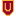Colorado State University-Pueblo

Gaussian Guesswork: Infinite Sequences And The Arithmetic-Geometric Mean, Janet Heine Barnett

Calculus

No abstract provided.

Evolution Of Superoscillations For Schrödinger Equation In A Uniform Magnetic Field, 2017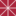Politecnico di Milano

Evolution Of Superoscillations For Schrödinger Equation In A Uniform Magnetic Field, Fabrizio Colombo, Jonathan Gantner, Daniele C. Struppa

Mathematics, Physics, and Computer Science Faculty Articles and Research

Aharonov-Berry superoscillations are band-limited functions that oscillate faster than their fastest Fourier component. Superoscillations appear in several fields of science and technology, such as Aharonov’s weak measurement in quantum mechanics, in optics, and in signal processing. An important issue is the study of the evolution of superoscillations using the Schrödinger equation when the initial datum is a weak value. Some superoscillatory functions are not square integrable, but they are real analytic functions that can be extended to entire holomorphic functions. This fact leads to the study of the continuity of a class of convolution operators acting on suitable spaces ...

Ar(1) Sequence With Random Coefficients:Regenerative Properties And Its Application, 2017Iowa State University

Ar(1) Sequence With Random Coefficients:Regenerative Properties And Its Application, Krishna B. Athreya, Koushik Saha, Radhendushka Srivastava

Communications on Stochastic Analysis

No abstract provided.

Constructing A Square An Ancient Indian Way Activity, 2017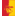Pittsburg State University

Constructing A Square An Ancient Indian Way Activity, Cynthia J. Huffman Ph.D.

Open Educational Resources - Math

In this activity students use string to model one of the ways that was used in ancient India for constructing a square. The construction was used in building a temporary fire altar. The activity is based on a translation by Sen and Bag of the Baudhāyana-śulba-sūtra.

Constructing A Square Indian Fire Altar Activity, 2017Pittsburg State University

Constructing A Square Indian Fire Altar Activity, Cynthia J. Huffman Ph.D.

Open Educational Resources - Math

In this activity, we will model constructing a square fire altar with a method similar to one used by people in ancient India. The fire altars, which were made of bricks, had various shapes. Instructions for building the altars were in Vedic texts called Śulba-sūtras. We will follow instructions for constructing a square gārhapatya fire altar from the Baudhāyana-śulba-sūtra, which was written during the Middle Vedic period, about 800-500 BC.

2017University of Wisconsin-Milwaukee

Splittings Of Relatively Hyperbolic Groups And Classifications Of 1-Dimensional Boundaries, Matthew Haulmark

Theses and Dissertations

In the first part of this dissertation, we show that the existence of non-parabolic local cut point in the relative (or Bowditch) boundary, $\relbndry$, of a relatively hyperbolic group $(\Gamma,\bbp)$ implies that $\Gamma$ splits over a $2$-ended subgroup. As a consequence we classify the homeomorphism type of the Bowditch boundary for the special case when the Bowditch boundary $\relbndry$ is one-dimensional and has no global cut points.

In the second part of this dissertation, We study local cut points in the boundary of CAT(0) groups with isolated flats. In particular the relationship between local cut points in ...

Extending Difference Of Votes Rules On Three Voting Models., 2017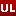University of Louisville

Extending Difference Of Votes Rules On Three Voting Models., Sarah Schulz King

Electronic Theses and Dissertations

In a voting situation where there are only two competing alternatives, simple majority rule outputs the alternatives with the most votes or declares a tie if both alternatives receive the same number of votes. For any non-negative integer k, the difference of votes rule Mk outputs the alternative that beats the competing alternative by more than k votes. Llamazares (2006) gives a characterization of the difference of votes rules in terms of five axioms. In this thesis, we extend Llamazares' result by completely describing the class of voting rules that satisfy only two out of his five axioms. In ...

2017University of Louisville

Residuated Maps, The Way-Below Relation, And Contractions On Probabilistic Metric Spaces., M. Ryan Luke

Electronic Theses and Dissertations

In this dissertation, we will examine residuated mappings on a function lattice and how they behave with respect to the way-below relation. In particular, which residuated $\phi$ has the property that $F$ is way-below $\phi(F)$ for $F$ in appropriate sets. We show the way-below relation describes the separation of two functions and how this corresponds to contraction mappings on probabilistic metric spaces. A new definition for contractions is considered using the way-below relation.

Dependence Structures In Lévy-Type Markov Processes, 2017University of Tennessee, Knoxville

Dependence Structures In Lévy-Type Markov Processes, Eddie Brendan Tu

Doctoral Dissertations

In this dissertation, we examine the positive and negative dependence of infinitely divisible distributions and Lévy-type Markov processes. Examples of infinitely divisible distributions include Poissonian distributions like compound Poisson and α-stable distributions. Examples of Lévy-type Markov processes include Lévy processes and Feller processes, which include a class of jump-diffusions, certain stochastic differential equations with Lévy noise, and subordinated Markov processes. Other examples of Lévy-type Markov processes are time-inhomogeneous Feller evolution systems (FES), which include additive processes. We will provide a tour of various forms of positive dependence, which include association, positive supermodular association (PSA), positive supermodular dependence (PSD), and positive ...

Π-Operators In Clifford Analysis And Its Applications, 2017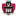University of Arkansas, Fayetteville

Π-Operators In Clifford Analysis And Its Applications, Wanqing Cheng

Theses and Dissertations

In this dissertation, we studies Π-operators in different spaces using Clifford algebras. This approach generalizes the Π-operator theory on the complex plane to higher dimensional spaces. It also allows us to investigate the existence of the solutions to Beltrami equations in different spaces.

Motivated by the form of the Π-operator on the complex plane, we first construct a Π-operator on a general Clifford-Hilbert module. It is shown that this operator is an L^2 isometry. Further, this can also be used for solving certain Beltrami equations when the Hilbert space is the L^2 space of a measure space. This ...

Vertex Weighted Spectral Clustering, 2017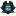East Tennessee State University

Vertex Weighted Spectral Clustering, Mohammad Masum

Electronic Theses and Dissertations

Spectral clustering is often used to partition a data set into a specified number of clusters. Both the unweighted and the vertex-weighted approaches use eigenvectors of the Laplacian matrix of a graph. Our focus is on using vertex-weighted methods to refine clustering of observations. An eigenvector corresponding with the second smallest eigenvalue of the Laplacian matrix of a graph is called a Fiedler vector. Coefficients of a Fiedler vector are used to partition vertices of a given graph into two clusters. A vertex of a graph is classified as unassociated if the Fiedler coefficient of the vertex is close to ...

2017Missouri State University

Cayley Graphs Of Groups And Their Applications, Anna Tripi

Cayley graphs are graphs associated to a group and a set of generators for that group (there is also an associated directed graph). The purpose of this study was to examine multiple examples of Cayley graphs through group theory, graph theory, and applications. We gave background material on groups and graphs and gave numerous examples of Cayley graphs and digraphs. This helped investigate the conjecture that the Cayley graph of any group (except Z_2) is hamiltonian. We found the conjecture to still be open. We found Cayley graphs and hamiltonian cycles could be applied to campanology (in particular, to the ...

2017Utah State University

Efficiently Representing The Integer Factorization Problem Using Binary Decision Diagrams, David Skidmore

All Graduate Plan B and other Reports

Let p be a prime positive integer and let α be a positive integer greater than 1. A method is given to reduce the problem of finding a nontrivial factorization of α to the problem of finding a solution to a system of modulo p polynomial congruences where each variable in the system is constrained to the set {0,...,p − 1}. In the case that p = 2 it is shown that each polynomial in the system can be represented by an ordered binary decision diagram with size less than 20.25log2(α)3 + 16.5log2(α)2 + 6log ...

Disciple, 2017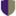Pacific Lutheran University

Disciple, Jessica K. Sklar

Journal of Humanistic Mathematics

This is a love poem for mathematics.

2017University of New South Wales

Discrete And Continuous: A Fundamental Dichotomy In Mathematics, James Franklin

Journal of Humanistic Mathematics

The distinction between the discrete and the continuous lies at the heart of mathematics. Discrete mathematics (arithmetic, algebra, combinatorics, graph theory, cryptography, logic) has a set of concepts, techniques, and application areas largely distinct from continuous mathematics (traditional geometry, calculus, most of functional analysis, differential equations, topology). The interaction between the two – for example in computer models of continuous systems such as fluid flow – is a central issue in the applicable mathematics of the last hundred years. This article explains the distinction and why it has proved to be one of the great organizing themes of mathematics.

Some Thoughts On The Epicurean Critique Of Mathematics, 2017American University of Kuwait

Some Thoughts On The Epicurean Critique Of Mathematics, Michael Aristidou

Journal of Humanistic Mathematics

In this paper, we give a comprehensive summary of the discussion on the Epicurean critique of mathematics and in particular of Euclid's geometry. We examine the methodological critique of the Epicureans on mathematics and we assess whether a 'mathematical atomism' was proposed, and its implications. Finally, we examine the Epicurean philosophical stance on mathematics and evaluate whether it was on target or not.